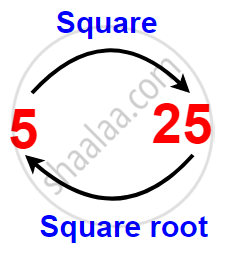# Concept of Square Roots

#### description

• Finding square roots

# Square Roots:

The inverse (opposite) operation of addition is subtraction and the inverse operation of multiplication is division. Similarly, finding the square root is the inverse operation of squaring.

We have,

122 =144, therefore square root of 144 is 12

92 = 81, therefore square root of 81 is 9.

Since 92 = 81, and (– 9)2 = 81

We say that square roots of 81 are 9 and – 9.

From the above, you may say that there are two integral square roots of a perfect square number.

The positive square root of a number is denoted by the symbol √.

For example: sqrt(4) = 2 ("not"  – 2); sqrt(9) = 3 ("not" – 3) etc.Statement Inference Statement Inference 12 = 1 sqrt(1) = 1 62 = 36 sqrt(36) = 6 22 = 4 sqrt(4) = 2 72 = 49 sqrt(49) = 7 32 = 9 sqrt(9) = 3 82 = 64 sqrt(64) = 8 42 = 16 sqrt(16) = 4 92 = 81 sqrt(81) = 9 52 = 25 sqrt(25) = 5 102 = 100 sqrt(100) = 10
If you would like to contribute notes or other learning material, please submit them using the button below.

### Shaalaa.com

What are Square Roots? [00:10:42]
S
0%